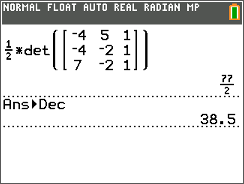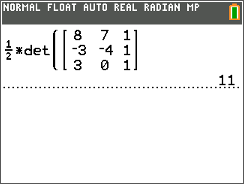# Activities

•##### Download

•• ##### Subject Area

• Math: Algebra II: Matrices

• ##### Author9-12

45 Minutes

• ##### Device
• TI-84 Plus
• TI-84 Plus Silver Edition
•TI-84 Plus C Silver Edition
•TI-84 Plus CE
• ##### Report an Issue

Triangle in the Matrix#### Activity Overview

Students represent a triangle with a matrix and use the determinant to find the area of three given triangles.

#### Key Steps

•Students will find the area of a right triangle using a matrix and verify the formula using A = 0.5bh.

•Students will practice using the matrix formula by finding the area of two non-right triangles using matrices.

In the extension, students are asked to find the vertex of a triangle given the other two vertices and the area.